Question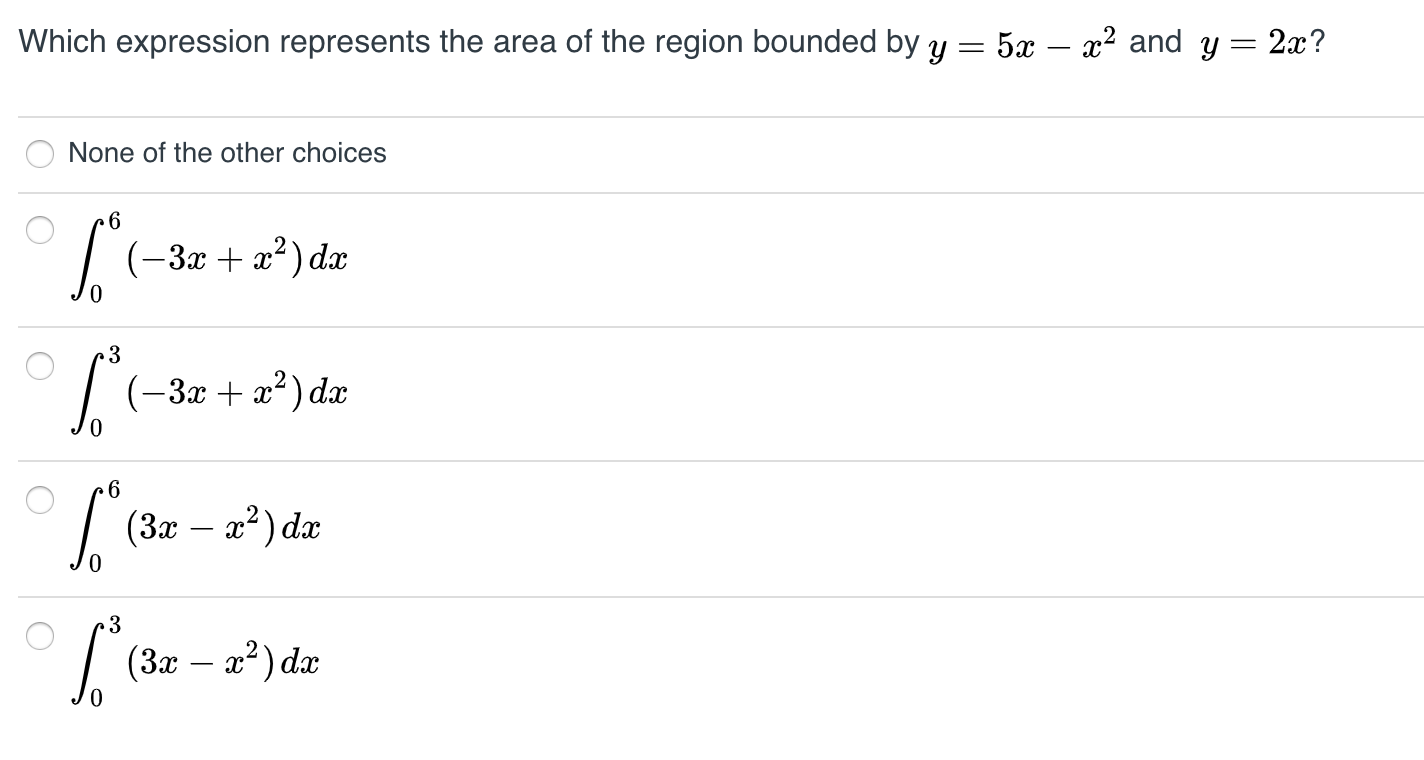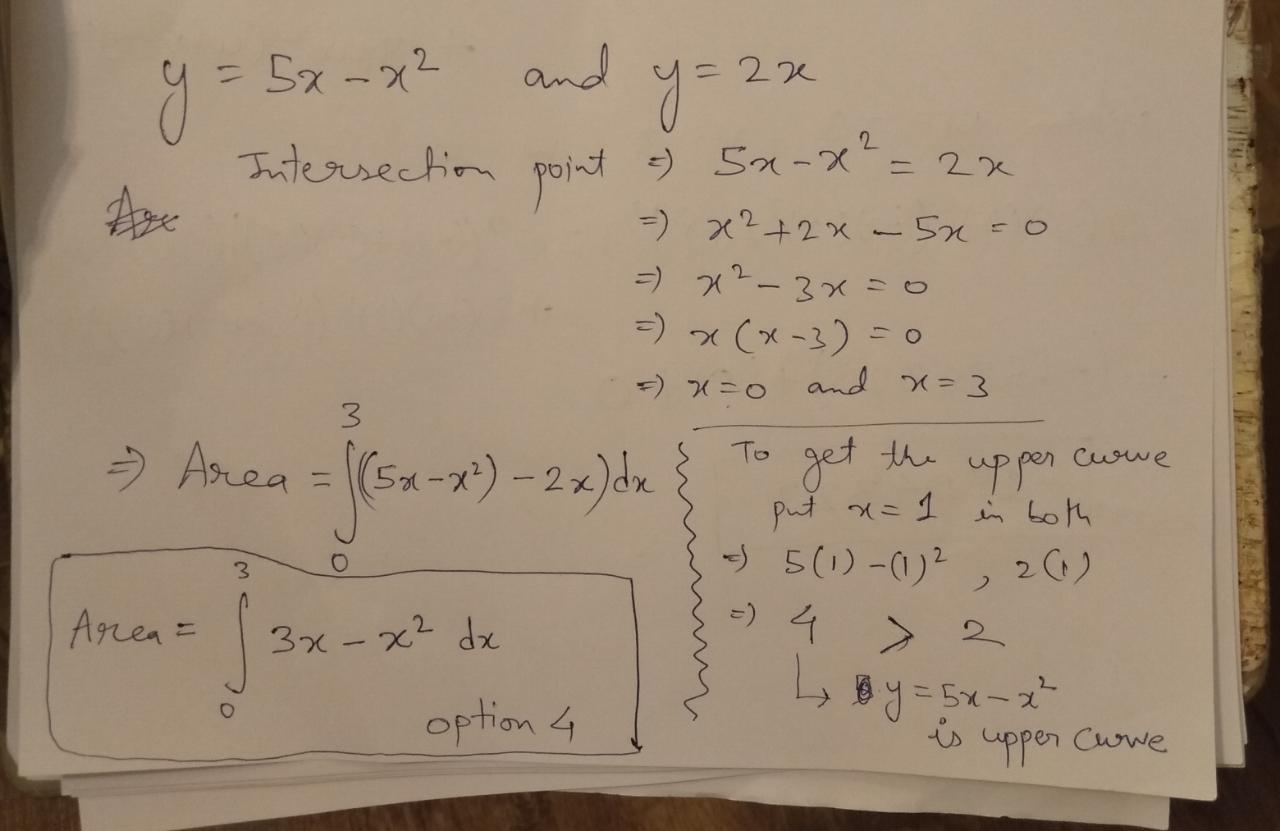#### Earn Coins

Coins can be redeemed for fabulous gifts.

Similar Homework Help Questions
• ### Question 25 Find the AREA of the region bounded by: y = x2 + 3x and...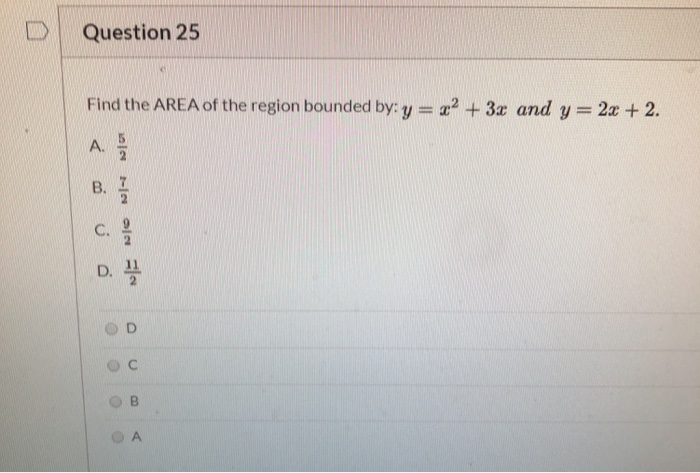Question 25 Find the AREA of the region bounded by: y = x2 + 3x and y=2x + 2. A. B. 2 C. Nowjo D. D

• ### Question 7 Using the method of disks/washers, which expression represents the volume of the solid obtained...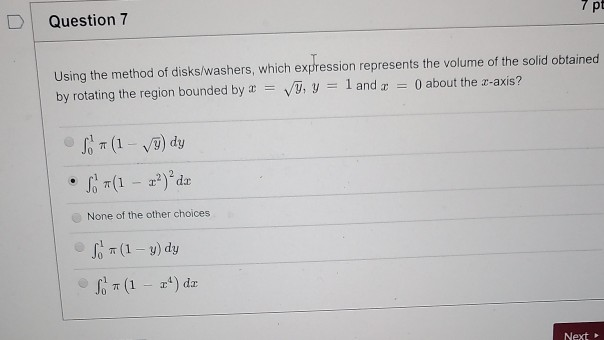Question 7 Using the method of disks/washers, which expression represents the volume of the solid obtained by rotating the region bounded by a = y, y = 1 and x = 0 about the z-axis? son (1 - ſ9) dy • Sor(1 – 2²) ² da None of the other choices S. (1 - y) dy S (1 – 24) de

• ### 4. 5. (a) Sketch the region bounded by y- +2x-4, y-x2+4x-4 clearly indicating vertices on the gra...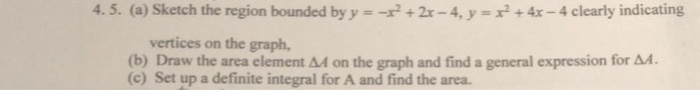4. 5. (a) Sketch the region bounded by y- +2x-4, y-x2+4x-4 clearly indicating vertices on the graph, (b) Draw the area element AA on the graph and find a general expression for A4. (c) Set up a definite integral for A and find the area. 4. 5. (a) Sketch the region bounded by y- +2x-4, y-x2+4x-4 clearly indicating vertices on the graph, (b) Draw the area element AA on the graph and find a general expression for A4. (c) Set...

• ### practice 1. Find the area of the region bounded by the curves. y= x2 - 4x,...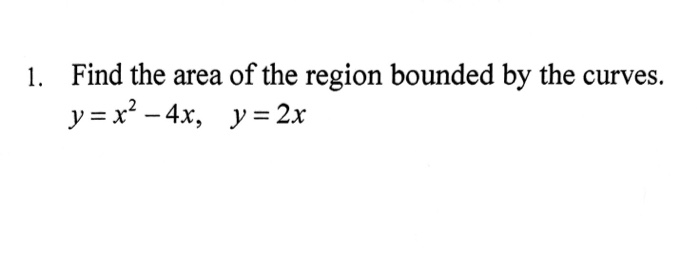practice 1. Find the area of the region bounded by the curves. y= x2 - 4x, y = 2x

• ### 2) The region R is bounded by the x-axis and y = V16 - x2 a)...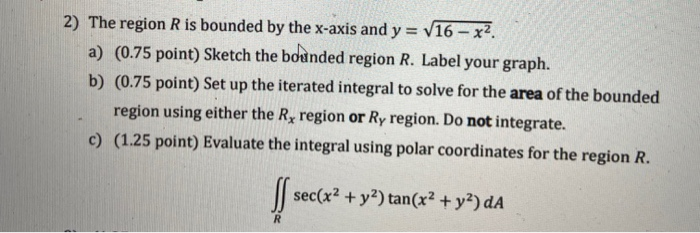2) The region R is bounded by the x-axis and y = V16 - x2 a) (0.75 point) Sketch the bounded region R. Label your graph. b) (0.75 point) Set up the iterated integral to solve for the area of the bounded region using either the Ry region or Ry region. Do not integrate. c) (1.25 point) Evaluate the integral using polar coordinates for the region R. sec(x2 + y2) tan(x2 + y2) dA R

• ### 2) The region R is bounded by the x-axis and y = V16 - x2 a)...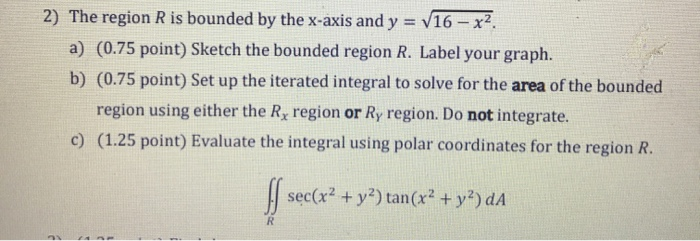2) The region R is bounded by the x-axis and y = V16 - x2 a) (0.75 point) Sketch the bounded region R. Label your graph. b) (0.75 point) Set up the iterated integral to solve for the area of the bounded region using either the Rx region or Ry region. Do not integrate. c) (1.25 point) Evaluate the integral using polar coordinates for the region R. sec(x2 + y2) tan(x2 + y2) dA R

• ### 2) The region R is bounded by the x-axis and y = V16 – x2. a)...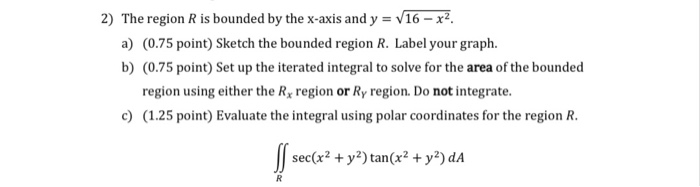2) The region R is bounded by the x-axis and y = V16 – x2. a) (0.75 point) Sketch the bounded region R. Label your graph. b) (0.75 point) Set up the iterated integral to solve for the area of the bounded region using either the Rx region or Ry region. Do not integrate. c) (1.25 point) Evaluate the integral using polar coordinates for the region R. S sec(x2 + y2) tan(x2 + y2) da R

• ### 2) The region R is bounded by the x-axis and y = V16 – x2 a)...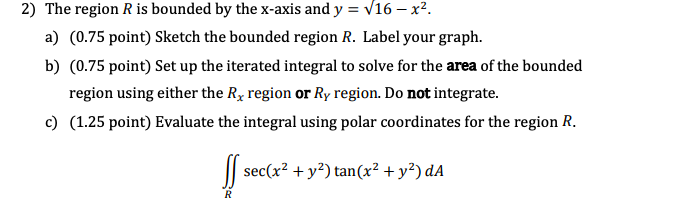2) The region R is bounded by the x-axis and y = V16 – x2 a) (0.75 point) Sketch the bounded region R. Label your graph. b) (0.75 point) Set up the iterated integral to solve for the area of the bounded region using either the Rx region or Ry region. Do not integrate. c) (1.25 point) Evaluate the integral using polar coordinates for the region R. S sec(x2 + y2) tan(x2 + y2) da R

• ### The region R is bounded by the x-axis and y = V16 – x2 a) Sketch...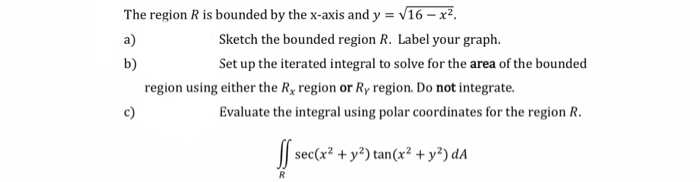The region R is bounded by the x-axis and y = V16 – x2 a) Sketch the bounded region R. Label your graph. b) Set up the iterated integral to solve for the area of the bounded region using either the Rx region or Ry region. Do not integrate. Evaluate the integral using polar coordinates for the region R. sec(x2 + y2) tan(x2 + y2) da c) R

• ### 2. Sketch the region bounded by the graphs of the equations and find the area of...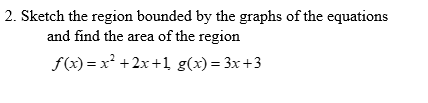2. Sketch the region bounded by the graphs of the equations and find the area of the region f(x) = x2 + 2x +1 g(x) = 3x +3